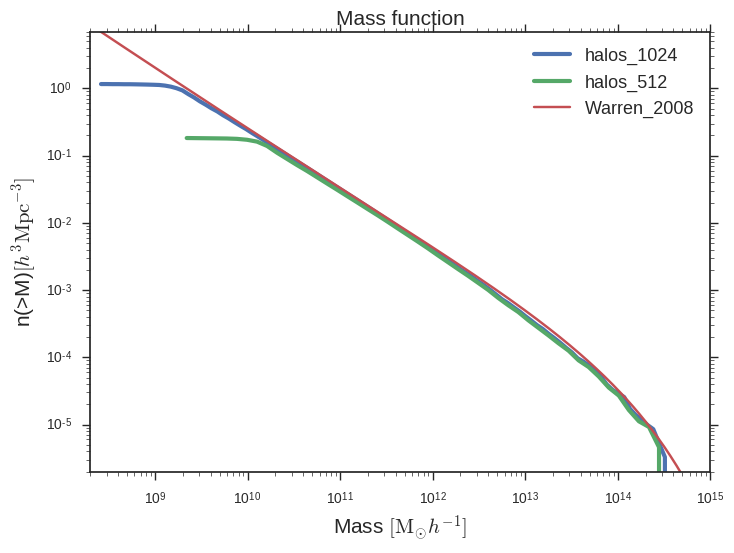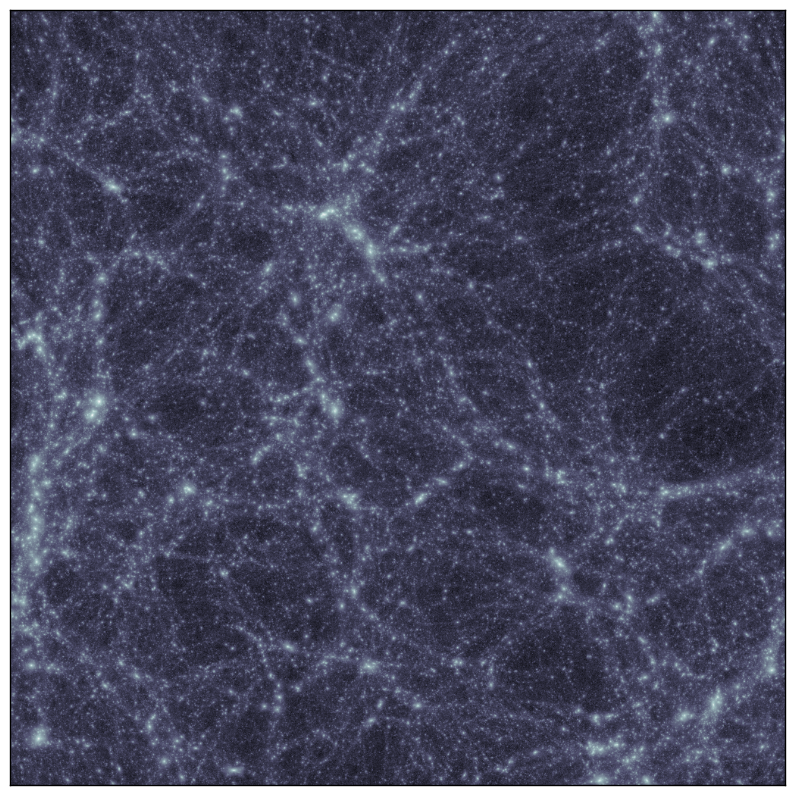SIMULATIONS PARAMETERS:

Box size: 115 Mpc/h

Cosmology from Plank (2016)Here

Cosmology:

• Omega_m = 0.3089

• Omega_L = 0.6911

• Omega_b = 0.0486

• H0 = 67.74

• sigma_8 = 0.8159

• nspec = 0.967

• transfer = eisenstein

Initial Conditions:

• Z = 100

• Power spectrum from Eisenstein & Hu transfer function

Force Resolution:

• 512: 4.9 kpc/h

• 1024: 2.4 kpc/h

• 2048: 1.2 kpc/h

Mass function at z=0 using ROCKSTAR halo catalogs for halo masses:Density projection using Brant’s code (image-snapshot):UPDATE

File with data for plotting mass functions from above Here

Script for plotting mass functions from data file Here

Data file: density projection Here

Data file: potential Here